• Index
•
• Previous
• Next
•
• Search

# 6.4   Probabilistic / Sensitivity Analysis

### Statistical Distributions

• The following types of statistical distributions can be assigned to various material and reinforcement parameters for probabilistic analysis. Material parameters include unit weight, cohesion, friction, ru, B-bar, and the strength with depth and anisotropic parameters. Reinforcement parameters include tensile, shear, and pullout resistance parameters, spacing, bond length, and area coverage.
• Note: All values generated by statistical distributions are limited to a lower bound value of 0 regardless.

• ## Normal Distribution

• The normal distribution takes the form of the "bell curve" shown below, and is defined by the mean (μ) and standard deviation (σ) of the random variable. Approximately 99.7% of values drawn from the normal distribution fall within 3 standard deviations of the mean.
• The normal distribution is limited in between the minimum (a) and maximum (b) values specified.

•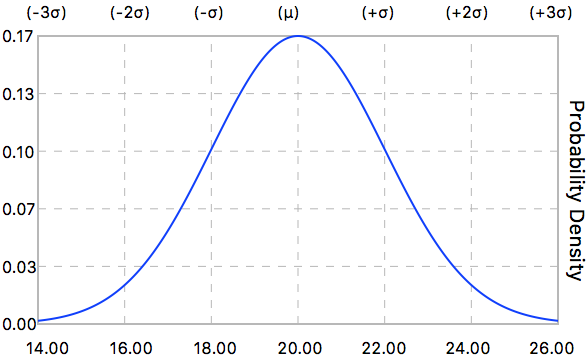• A random value (X) is generated from the normal distribution using the following equation:

•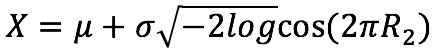## Log-Normal Distribution

• The log-normal distribution is defined for a random variable whose logarithm is normally distributed. It can be useful to model random variables (such as cohesion and pore pressure) which may have a peak in probability density near zero and gradually taper off for larger values. It is defined by the mean (μ) and standard deviation (σ) of the random variable.
• The log-normal distribution is limited in between the minimum (a) and maximum (b) values specified.

•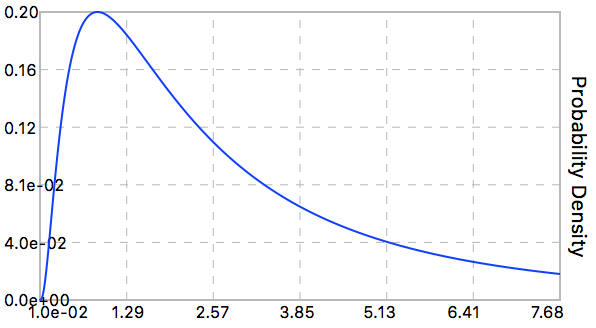• A random value (X) is generated from the log-normal distribution using the following equation:

•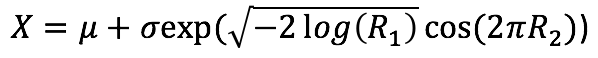## Uniform Distribution

• The uniform distribution takes the form of the rectangle shown below, and is defined by a lower value 'a' and upper value 'b', where all values within this range are equally probable. The probability density is defined as 1 / (b - a).

•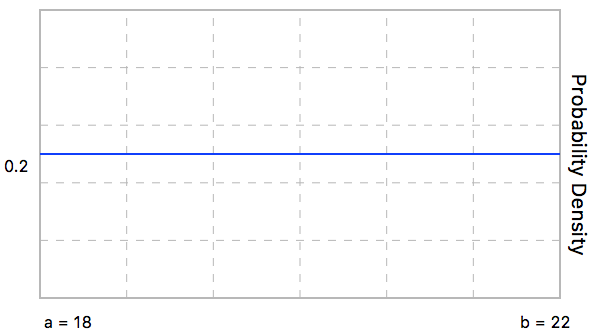• A random value (X) is generated from the uniform distribution using the following equation:

•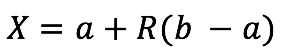### Monte Carlo Analysis

• After a standard analysis has been completed using deterministic material parameters, a Monte Carlo probabilistic analysis can be completed for the current selected trial by selecting Probabilistic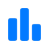from the analysis results table.
• The Monte Carlo analysis generates and calculates the FOS for the specified # of Trials using the currently selected slip surface. For each trial unique values for every random variable are generated from their associated statistical distributions.
• The probability that the FOS is less than a specified value is defined as follows, where x is specified by the user:
•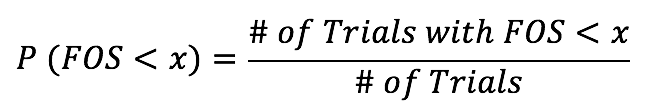• Note: Parameters with theicon activated (in blue) in the material / reinforcement editors are included as random variables in the probabilistic analysis. All other parameters are maintained at their deterministic values for the analysis.
• Once the calculations are complete, select FOS Probability Distribution from the Probabilistic menu to view the distribution of FOS calculated for the random variables.

• ### Sensitivity Analysis

• After a standard analysis has been completed using deterministic material parameters, a sensitivity analysis can be completed for the current selected trial by selecting Sensitivity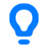from the analysis results table.
• A sensitivity analysis is essentially a Monte Carlo probabilistic analysis, however only the single Variable specified is altered, while all others are maintained at their deterministic values.
• The sensitivity analysis generates and calculates the FOS for the specified # of Trials using the currently selected slip surface. For each trial unique values for the selected random variable are generated from the associated statistical distribution.
• Once the calculations are complete, select FOS Sensitivity Plot from the Sensitivitymenu to view the distribution of FOS calculated for the specified variable.
• Specifying the Sensitivity FOS highlights the value of the sensitivity variable that corresponds to that FOS in the FOS Sensitivity Plot.